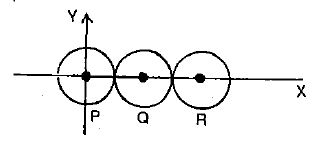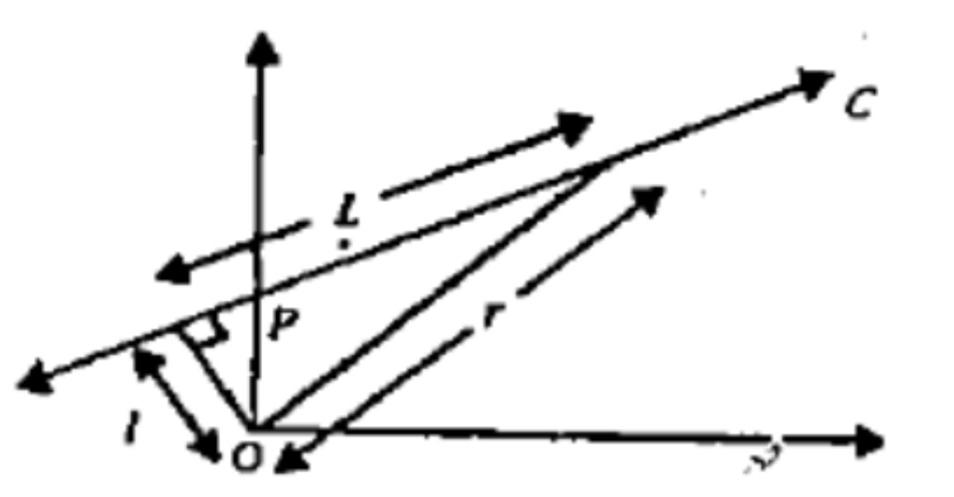A loop rolls down on the inclined plane. The fraction of its total kinetic energy that is associated with rotational motion is

1.  1: 2

2.  1: 3

3.  1: 4

4.  2: 3

Concept Questions :-

Rolling motion
High Yielding Test Series + Question Bank - NEET 2020

Difficulty Level:

A ball kept in a closed box moves in the box making collisions with the walls. The box is kept on a smooth surface. The velocity of the centre of mass

1. of the box remains constant

2. of the box plus the ball system remains constant

3. of the ball remains constant

4. of the ball relative to the box remains constant

Concept Questions :-

Center of mass
High Yielding Test Series + Question Bank - NEET 2020

Difficulty Level:

A body of mass ${\mathrm{m}}_{1}$ moving with a velocity  collides elastically with another body  at rest of mass ${\mathrm{m}}_{2}$. After collision the velocities of the two bodies are  and  respectively along the direction of motion of ${\mathrm{m}}_{2}$. The ratio $\frac{{\mathrm{m}}_{1}}{{\mathrm{m}}_{2}}$ is

1.  $\frac{5}{12}$

2.  $5$

3.  $\frac{1}{5}$

4.  $\frac{12}{5}$

Concept Questions :-

Linear momentum
High Yielding Test Series + Question Bank - NEET 2020

Difficulty Level:

The M.I. of a body about the given axis is 1.2 kg x ${\mathrm{m}}^{2}$ initially the body at rest. In order to the rotational kinetic energy of 1500 J, the angular acceleration of 25 rad/${\mathrm{sec}}^{2}$ must be about that axis for the duration of

1.  4 sec

2.  2 sec

3.  8 sec

4.  10 sec

Concept Questions :-

Rotation motion : Introduction
High Yielding Test Series + Question Bank - NEET 2020

Difficulty Level:

Two particles of equal mass have coordinates (2m,4m,6m) and (6m,2m,8m). Of these one particle has velocity ${\mathrm{v}}_{1}=\left(2\stackrel{^}{\mathrm{i}}\right)$ m/s and another particle has velocity ${\mathrm{v}}_{2}=\left(2\stackrel{^}{j}\right)$ m/s at time t = 0. The coordinate of their centre of mass at time t = 1 s will be

1. (4m,4m,7m)

2. (5m,4m,7m)

3. (2m,4m,6m)

4. (4m,5m,4m)

Concept Questions :-

Center of mass
High Yielding Test Series + Question Bank - NEET 2020

Difficulty Level:

Three indentical spheres, each of mass 1 kg are kept as shown in figure, touching each other, with their centres on a straight line. If their centres are marked P, Q, R respectively, the distance of centre of mass of the system from P isConcept Questions :-

Center of mass
High Yielding Test Series + Question Bank - NEET 2020

Difficulty Level:

A mass m with velocity u strikes a wall normally and returns at the same speed. What is the magnitude of the change in momentum of the body when it returns

1.  4 mu

2.  mu

3.  2 mu

4.  0

Concept Questions :-

Linear momentum
High Yielding Test Series + Question Bank - NEET 2020

Difficulty Level:

Two bodies with moment of inertia  $\left({I}_{1}>{I}_{2}\right)$ and have equal angular momenta.  If KE of rotation are , then

(1) ${E}_{1}={E}_{2}$                (2) ${E}_{1}>{E}_{2}$                (3)  ${E}_{1}<{E}_{2}$              (4) ${E}_{1}\ge {E}_{2}$

High Yielding Test Series + Question Bank - NEET 2020

Difficulty Level:

A particle of mass m moves along line PC with velocity v as shown.  What is the angular momentum of the particle about P?(1) mvL                 (2) mvl                (3) mvr                  (4) zero

(1) $\frac{R}{\left(4\mathrm{\pi }-2\right)}$             (2) $\frac{R}{2\mathrm{\pi }}$                (3) $\frac{R}{\left(\mathrm{\pi }-2\right)}$                     (4) $\frac{R}{\left(2\mathrm{\pi }-2\right)}$